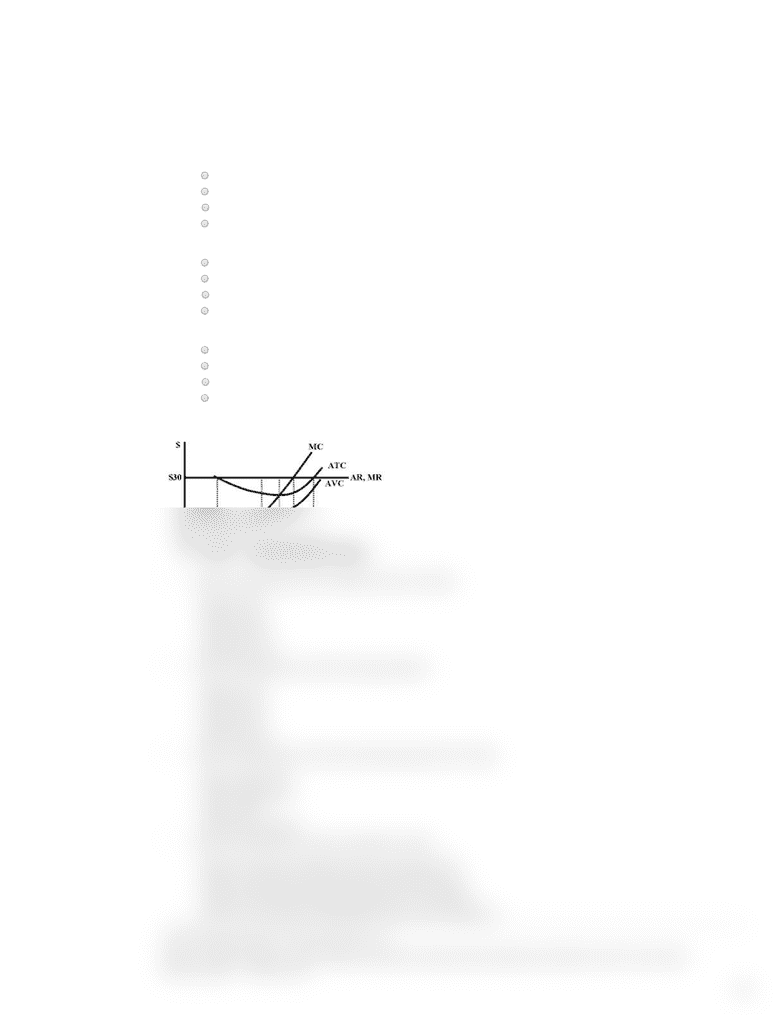# ECON 1 Study Guide - Midterm Guide: Perfect Competition, Demand Curve

102 views2 pages1/2
Chapter 7
1 Which of the following is not one of the characteristics of a perfectly competitive market?
A) Firms sell differentiated products
B) Productive resources are mobile
C) Buyers and sellers are well informed
D) The market has many buyers and sellers, each of which buys or sells only a small fraction of the total quantity exchanged
2 The demand curve of an individual firm in a perfectly competitive market is
A) Perfectly inelastic
B) Perfectly elastic
C) Unit elastic
D) None of the above
3 If a firm would like to stay in the market in the long run, then
A) It needs to cover its variable costs
B) It needs to cover its explicit costs
C) It needs to cover its fixed costs
D) It needs to cover all costs
Use the graph below to answer questions 4 to 7
4 Based on the graph above, the firm breaks even when it produces:
A) 200 units
B) 700 units
C) 1000 units
D) Both a and c
5 Based on the graph above, the profit maximizing quantity is
A) 500 units
B) 700 units
C) 850 units
D) 1000 units
6 Based on the graph above, at the profit maximizing quantity, the firm makes
A) A positive profit
B) A negative profit
C) No profit
D) None of the above
7 Based on the graph above, the long run equilibrium involves
A) Firms leaving the market and economic profit rising to zero
B) Firms entering the market and economic profit falling to zero
C) No firms entering the market because the firm is a monopolist
D) No firms entering the market because economic profit is already zero
Use the following information to answer questions 8 to 11
Based on marketing research, a company obtains the following information about the demand and production costs of its new product:
P = 800 – 20Q
MC = 80 + 20Q
Unlock document

This preview shows half of the first page of the document.
Unlock all 2 pages and 3 million more documents.

## Document Summary

The demand curve of an individual firm in a perfectly competitive market is: perfectly inelastic, perfectly elastic, unit elastic, none of the above. Use the graph below to answer questions 4 to 7. Based on the graph above, the firm breaks even when it produces: 200 units, 700 units, 1000 units, both a and c. Based on the graph above, the profit maximizing quantity is: 500 units, 700 units, 850 units, 1000 units. Based on the graph above, at the profit maximizing quantity, the firm makes: a positive profit, a negative profit, no profit, none of the above. Use the following information to answer questions 8 to 11. Based on marketing research, a company obtains the following information about the demand and production costs of its new product: The quantity that maximizes the company"s profit is: 10, 12, 18, 20. Following question 9, the price that maximizes the company"s profits is: 400, 440, 500, 560.